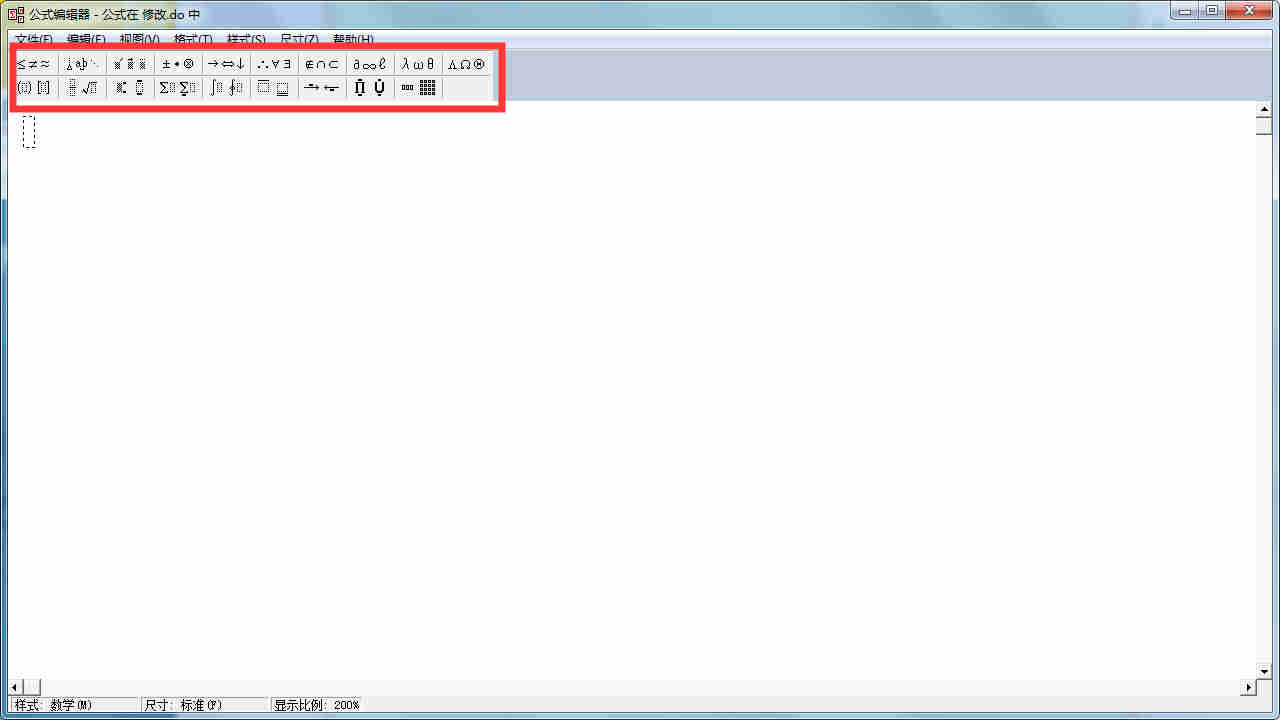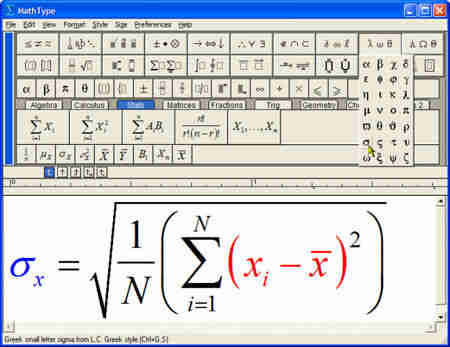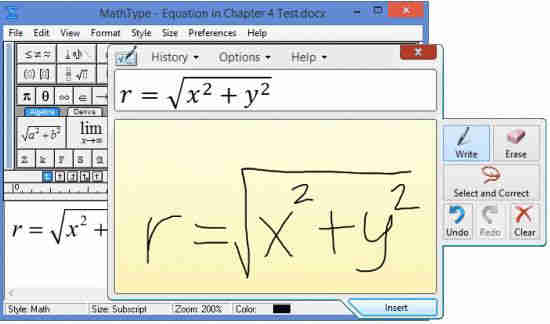### word中公式输入技巧有哪些？1. 快捷键

(1) 插入常用符号

2.批量修改公式的字号和大小

(1)双击一个公式，打开MathType，进入编辑状态；

(2)点击size菜单——define——字号对应的pt值，一般五号对应10pt，小四对应12pt

(3)点击preference->equation preference -> save to file ->存一个与默认配置文件不同的名字；

(4)关闭MathType回到word文档；

3.手写输入数学标记\x^3x3x3            \x_3x3x3

\sum∑∑            \sum_a^bNi=1i=1N

\int∫∫            \int_a^bbaab

\iint∬∬            \iint_a^bbaab

\prod∏∏            \prod_{i=1}^NNi=1i=1N

\bigcup⋃⋃            \bigcup_{i=1}^NNi=1i=1N

\bigcap⋂⋂            \bigcap_{i=1}^NNi=1i=1N

\lim_{x \to 0}limx0limx0            \frac{1}{2}1212

\sqrt{2}2–√2            \sqrt{2}2–√323

2020-01-06 17:22:02